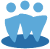## Section 1 of the SSG in Toolkit 6

This page covers several smaller questions that we have seen about Section 1 (The Front Page) of the Stock Study in Toolkit 6.

Q: How does Toolkit calculate PTP numbers? I don't see where they are input into the Annual Data and the Quarterly Data numbers do not always add up to the Annual number. Is there a calculation involved using other numbers in the Data page?

A: The data includes annual Net Profit and the Tax Rate. Toolkit calculates pre-tax profit from those (i.e., multiply the net profit by the “not-tax” rate).
The quarterly and annual figures are separate, so they may not add up precisely, because of rounding or because of normalization that is done on the annual figures.

Q: The % Institutions and % Insiders figures add up to more than 100%, is there something wrong with the data?

A: Not necessarily; by Morningstar's standards, any institution that owns more than 5% of a company's stock is also classified as an insider. This means that those two figures can end up higher than 100%.

Q: How does Toolkit figure out Debt to Capital?

A: Debt to capital in Toolkit is [Last Quarterly Total Debt] / [Total Capital

• Last Quarterly Total Debt is calculated as [Last Quarterly Short Term Debt (Commercial Paper + Current Portion of Long-Term Debt)] + [Last Quarterly Long Term Debt]
• Total Capital is [Last Quarterly Shareholder’s Equity] + [Last Quarterly Total Debt]

In cases where a Total Capital figure is not available (such as in preliminary data), Toolkit will calculate it as follows:

[Last Quarterly Total Debt] / [Last Quarterly Total Debt + Last Quarterly Preferred Stock + (Last Annual Book Value per Share * Last Quarterly Diluted Shares))

Q: What is Free Cash Flow?

A: For an explanation of Free Cash Flow, we suggest an article by Carol Theine in the Puget Sound Chapter’s newsletter about cash flow analysis basics.